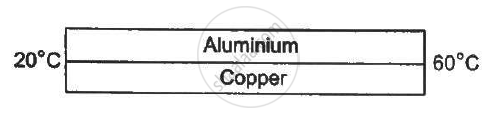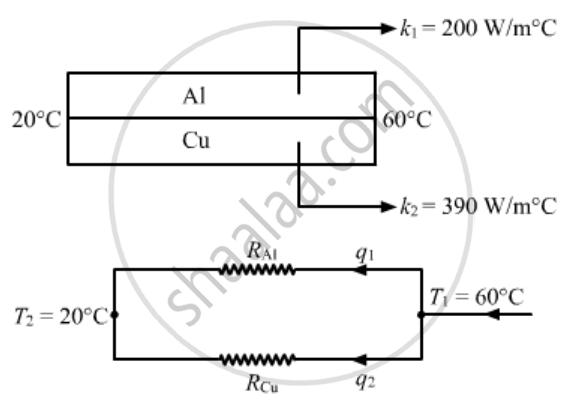Share

# An Aluminium Rod and a Copper Rod of Equal Length 1.0 M and Cross-sectional Area 1 Cm2 Are Welded Together as Shown in Figure (28−E3). One End is Kept at a Temperature of 20°C and the Other at - Physics

ConceptThermal Expansion of Solids

#### Question

An aluminium rod and a copper rod of equal length 1.0 m and cross-sectional area 1 cm2 are welded together as shown in the figure . One end is kept at a temperature of 20°C and the other at 60°C. Calculate the amount of heat taken out per second from the hot end. Thermal conductivity of aluminium = 200 W m−1°C−1 and of copper = 390 W m−1°C−1.#### Solutionq1 and q2 are heat currents. In other words, they are the rates of flow of heat through aluminium and copper rod, respectively.

Applying KVL at the hot junction, we get

q = q1 + q2

Rate of heat flow, q  = (KA Delta T)/ l

As q = q1 + q2

(K_pA(T_1 + T_2))/l = (k_1A(T_1 - T_2))/l +(KA Delta T)/ l

Kp =K1 + k2

= 390 + 200 = 590 W/m°C

⇒ q = (K_PA( T_1 _ T_2))/l

q= 2.36 W

Is there an error in this question or solution?

#### APPEARS IN

Solution An Aluminium Rod and a Copper Rod of Equal Length 1.0 M and Cross-sectional Area 1 Cm2 Are Welded Together as Shown in Figure (28−E3). One End is Kept at a Temperature of 20°C and the Other at Concept: Thermal Expansion of Solids.
S